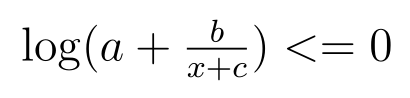# A common expression but how to transmit it into a CVX expression?As shown in the picture, the parameters of a,b,c are positive contants. Obviously, the expression is convex, but how to directly write it in CVX form? And I mean that we should not apply any slack conditions or the successive approximation method.

Many papers said this expression is convex and can be solved by optimization tools. I do not know how to directly solve it with CVX, who can tell me how to solve this? Thanks!

It is convex presuming a, b, c are all nonnegative. Have you tried to adapt the solution by @Michal_Adamaszek to your previous problem CVX wrong. How can I deal with this problem correctly? ?

I suppose you can do

a+\frac{b}{x+c} \leq 1

or? And that can be reduced to a linear expression if x is nonnegative.

Thanks. I guess your method is feasible. However, I still want to know if the expression is changed into \log(a+\frac{b}{x+c})\leq y, with y>0. Is there any method to solve this in CVX form?

Thanks a lot. I have gotten the feasible solution in terms of your instruction.

You should be able to use the

\ln\left (1+\frac{1}{x} \right ) \leq t

idea from

Thank you. If possible, can you show me more details on how to write this constraint in CVX code based on Conic method. Because I still feel confused to the PDF attachment.

Using the simple rewrite

\ln \left (a+\frac{1}{z}\right ) \leq t

and

z = (c + x)/b \geq 0.

If

a=1

then you have the case in the cheat sheet. It should be easy to adapt the idea from the cheat sheet to more general a.

The exponential cone and rotated quadratic cone required by cheat sheet solution can be handled by Cvx, but it might be easier and more efficient to use Mosek directly.

There might also be another way of doing that fits Cvx better but at least you know one way now.